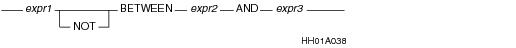# 15.00 - BETWEEN / NOT BETWEEN - Teradata Database

## Teradata Database SQL Functions, Operators, Expressions, and Predicates

Product
Release Number
15.00
Content Type
Programming Reference
Publication ID
B035-1145-015K
Language
English (United States)

## BETWEEN / NOT BETWEEN

### Purpose

Tests whether an expression value is between two other expression values.

### Syntax### ANSI Compliance

BETWEEN and NOT BETWEEN are ANSI SQL:2011 compliant.

### Usage Notes

The BETWEEN test is satisfied if the following condition is true.

`   expression_2 <= expression_1 <= expression_3 `

If the BETWEEN test fails, no rows are returned.

The BETWEEN test is treated as two separate logical comparisons.

`   expression_1 >= expression_2 AND expression_1 <= expression_3. `

 This expression … Is equivalent to … x BETWEEN y AND z ((x >= y) AND (x <=z))

Note that because expression_1 is actually evaluated twice, using a nondeterministic function, such as RANDOM, can produce unexpected results.

### Example

The following example uses a search condition in a HAVING clause to select from the Employee table those departments with the number 100, 300, 500, or 600, and with a salary average of at least \$35,000 but not more than \$55,000:

`   SELECT AVG(Salary) `
`   FROM Employee `
`   WHERE DeptNo IN (100,300,500,600) `
`   GROUP BY DeptNo `
`   HAVING AVG(Salary) BETWEEN 35000 AND 55000 ;`